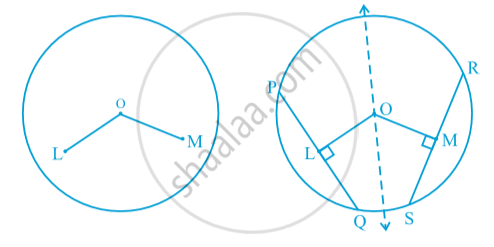# Equal Chords and Their Distances from the Centre

#### theorem

Theorem: Equal chords of a circle (or of congruent circles) are equidistant from the centre (or centres).
Consider a circle of centre O . It has PQ and RS two chords of equal length.Now , draw a perpendicular from centre to PQ and RS intersecting them at L and M .
To Prove: OL = OM
Join OQ and OS.
Proof:  We know that PQ =RS  (given)
QL = (PQ )/2 (perpendicular drawn from a centre to a chord bisect the chord)
Similarly, SM =(RS)/2
Therefore , QL = SM
Consider ∆OQL and ∆OSM
OQ = OS  (radius of the same circle)
QL = SM (proved)
∠OLQ = ∠OMS = 90°  (given )
∆OQL ≅ ∆OSM    (SSA rule)
OL = OM (By CPCT).

Theorem: Chords equidistant from the centre of a circle are equal in length.

If you would like to contribute notes or other learning material, please submit them using the button below.

### Shaalaa.com

Theorem: Equal chords of a circle (or of congruent circles) are equidistant from the centre (or centres). [00:05:28]
S
0%